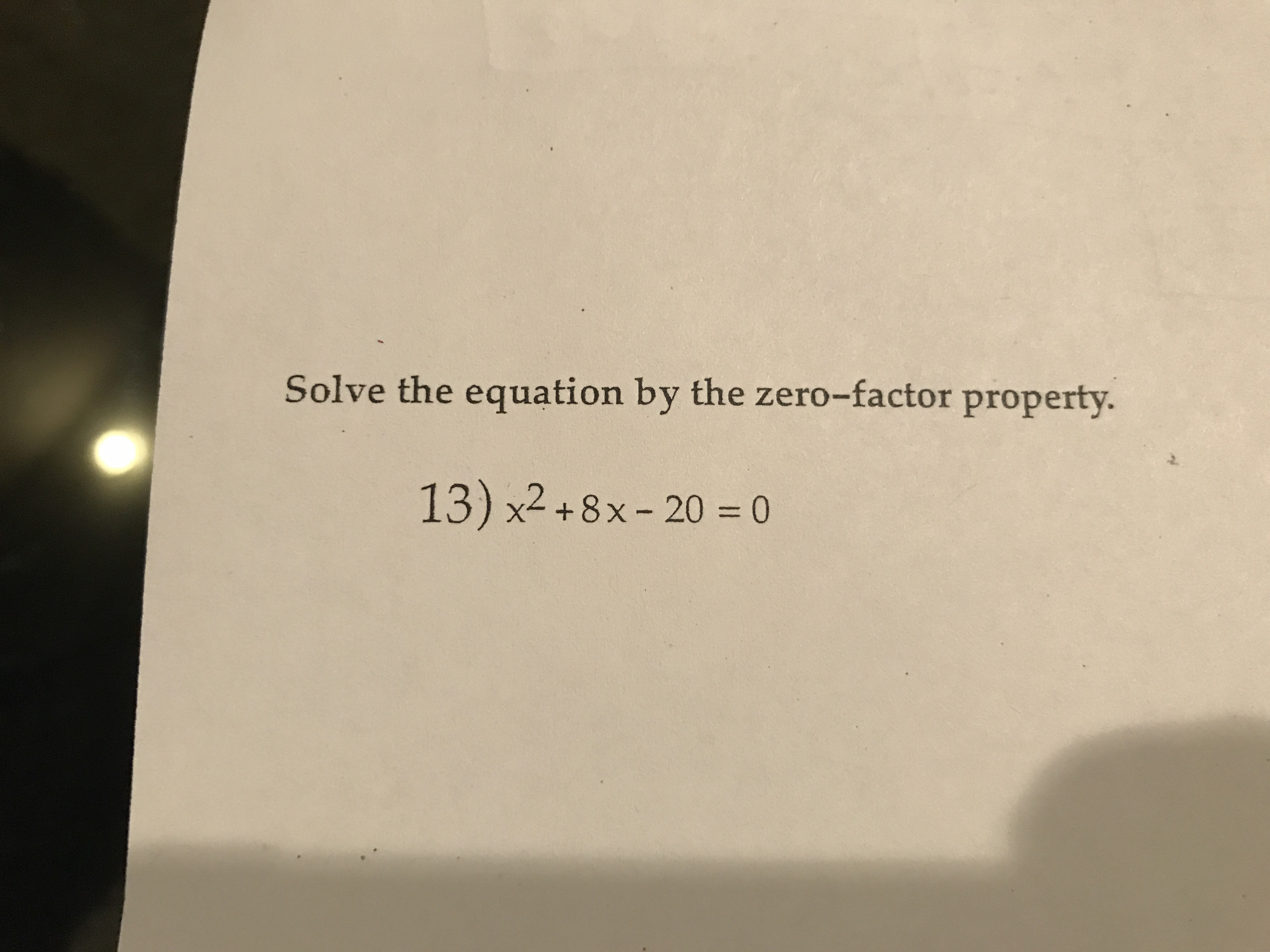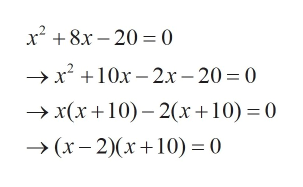Solve the equation by the zero-factor property.13) x2+8x-20 = 0

Questionhelp_outlineImage TranscriptioncloseSolve the equation by the zero-factor property. 13) x2+8x-20 = 0 fullscreen
Step 1

Given equation is

Step 2

Zero factor property: If (x+a)(x+b)=0 then x+a=0 or x+b=0

Step 3

Factorise the give...help_outlineImage Transcriptionclosex28x-20 0 -x 10x 2x- 20 0 x(x10) 2(x+10) 0 (x-2)x10)0 fullscreen

Want to see the full answer?

See Solution

Want to see this answer and more?

Our solutions are written by experts, many with advanced degrees, and available 24/7

See Solution
Tagged in

Equations and In-equations